Study of mathematics online.
Study math with us and make sure that "Mathematics is easy!"

# Circle, disk, segment, sector. Formulas, characterizations and properties of circle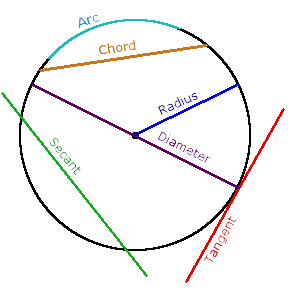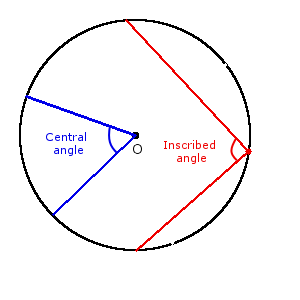Definition. Circle is a set of all points in the plane which are equidistant from a given point О, called the center of circle.
Definition. Unit circle is a circle, whose radius is equal to one.
Definition. Disk is part of the plane bounded by circle.
Definition. Radius of circle R is the distance from the circle center О to any point on the circle.
Definition. Diameter of circle D is a segment that connects two points on circle and passes through its center.

## Properties of a circle

1. Diameter of circle is equal two radiuses.

D = 2r

2. The shortest distance from the center circle to the secant (chord) is always smaller radius.
3. Three points that not placed on a straight line can hold only one circle.
4. Among all closed curves of equal length, circle has the largest area.
5. If two circle touch at one point, this point placed on the line that passes through the centers of the circles.

## Area and circumference of circle

### Length of circumference

1. Formula of the circumference length in terms of the diameter:

C = πD

2. Formula of the circumference length in terms of the radius:

C = 2πr

### Formula of the circle area

1. Formula of the circle area in terms of the radius:

A = πr2

2. Formula of the circle area in terms of the diameter:

A = πD24

## The equation of circle

1. The equation of circle with radius r and center at the start of Cartesian coordinate:

r2 = x2 + y2

2. The equation of circle with radius r and center at point with coordinates (a, b) in the Cartesian coordinate:

r2 = (x - a)2 + (y - b)2

3. Parametric equations of circle with a radius r and center at point with coordinates (a, b) in the Cartesian coordinate:
 { x = a + r cos t y = b + r sin t

## Tangent of circle and its properties

Definition. Tangent is a coplanar straight line that touches the circle at a single point.

## Tangent properties

1. Tangent is always perpendicular to the radius of the circle drawn at the point of contact.
2. The shortest distance from the center of circle to tangent is radius of the circle.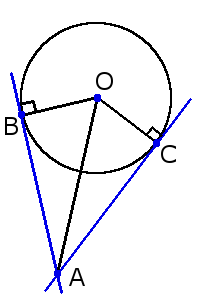3. If two tangents from touch points B and C on the same circle are not parallel, they intersect at point A and the point of contact between the segment and the point of intersection of a tangent is the same segment on a different tangent:

AB = AC

Also, if you draw a line through the center O and the intersection point A of these tangents, the angles between this line and tangents will be equal:

∠ОAС = ∠OAB

## Secant of circle and its properties

Definition. Secant of a circle is a straight line, connecting two points of circle.

### Properties of circle secant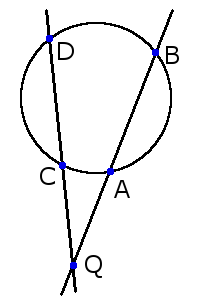1. If a point outside the circle (Q) obtained two secant, crossing the circle at two points A and B for a first secant and C and D for another secant, the products of two intersecting segments are equal:

AQ ∙ BQ = CQ ∙ DQ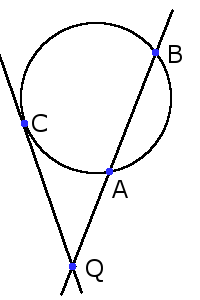2. If the point comes out of Q circle secant, crossing the circle at two points A and B, and the tangent point of contact C, then product segments the secant lengths equal to the square of the tangent:

AQ ∙ BQ = CQ2

## Chord of circle and its properties

Definition. Chord of a circle is a segment that connects two points of circle. Chord is a segment of tangent.

### Length of chord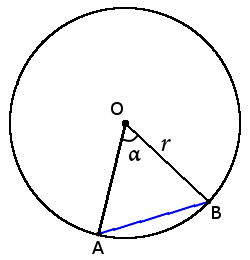1. Formula of the chord length in terms of the radius and central angle:

AB = 2r sin α22. Formula of the chord length in terms of the radius and inscribed angle:

AB = 2r sin α

### Chord properties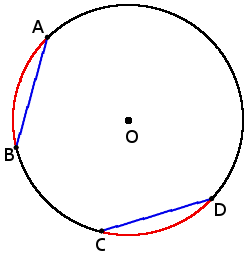1. Two equal chords tightening two identical arc:

if chords AB = CD, then

arc ◡ AB = ◡ CD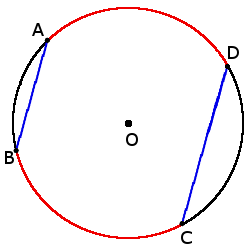2. If the chord are parallel, the arcs between them will be the same:

if chords AB ∣∣ CD, then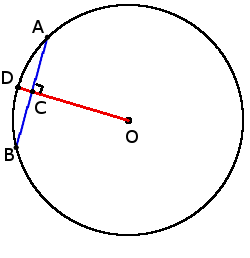3. If radius of circle is perpendicular to the chord, it divides chord in half at the point of intersection:

if OD AB, then

AC = BC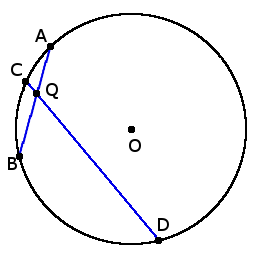4. If two chords AB and DE intersect at point Q, then the product segments, formed at the intersection, one chord is the product of a different chord sections:

AQ ∙ BQ = DQ ∙ QC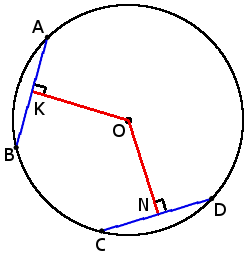5. Chords of equal length are equidistant from the center of circle.

if chords AB = CD, then

ON = OK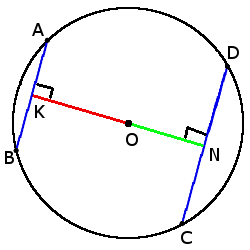6. The greater chord the closer it is to the center.

if CD > AB, then

ON < OK

## Central angle and inscribed angle of a circle and its properties

Definition. Central angle circle is the angle, the apex of which is the center of circle.
Definition. Inscribed angle is the angle inside the circle, the apex of which lies on the circle.

## Angle properties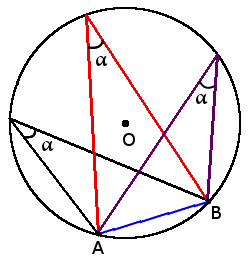1. All inscribed angles, based on one arc is equal (one end of the chord).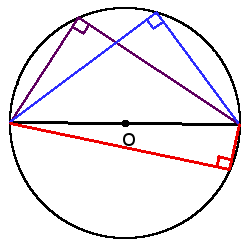2. Inscribed angle will be a straight (90°), if it is based on the diameter of the circle.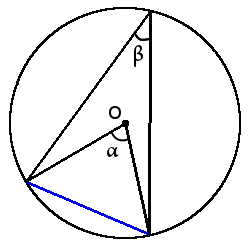3. Any inscribed angle is always equal to half the central angle, based on the same arc

β = α2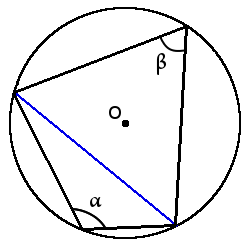4. If two inscribed angles based on a chord and located on either side of her, the sum of the angles is 180°.

α + β = 180°

Definition. Arc of a circle (◡) is part of the circle, connecting two points on the circle.
Definition. Subtending angle of an arc is angle between two radii which limit this arc. Subtending angle arc is always equal the central angle between radii, which limits end points this arc.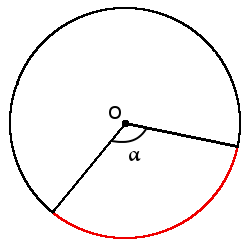Formula of the arc length in terms of the central angles (in degrees) and radius:

l = πr180°∙ α

Definition. Semicircle is arc whose ends are connected by diameter of a circle.
Definition. Semidisc () is part of the disk, which is bounded by a semicircle and diameter.
Definition. Sector () is part of the disk, which is bounded by two radii and an arc between the radii.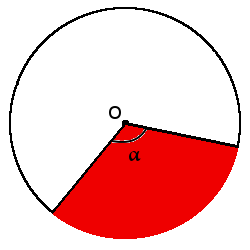Formula. Formula of the sector area in terms of the radius and central angles (in degrees)

A = πr2360°∙ α

Definition. Segment is part of the disk, which is bounded by the arc and the chord connecting the ends of this arc.
Definition. Concentric circle is circle with different radii whish having a common center.
Definition. Annulus is part of the plane bounded by two concentric circles.